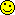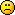<![CDATA[Math Is Fun Forum / find area]]> 2021-02-20T01:32:00Z FluxBB http://www.mathisfunforum.com/viewtopic.php?id=26016 <![CDATA[Re: find area]]> Now knowing the radius, here are 2 methods for finding the area of the shaded region:

In the formulas...
r = the radius of the circumscribed circle
i = the radius of the respective inscribed circle
s = the sagitta of the respective circular segment

Method A (Area of circular segments minus area of inscribed circles)
1. Total area of the 3 circular segments = 481.49203......respective segment areas = r²ArcCos((r - s)/r) - (r - s)√(2rs - s²)
2. Total area of the 3 inscribed circles R, M & L = 175.92919......respective circle areas = πi²

481.49203
- 175.92919
------------
=======

Method B (Area of circumscribed circle minus areas of inscribed objects)
1. Area of circumscribed circle = 756.47691......πr²
2. Total area of the 3 inscribed circles R, M & L = 175.92919......respective circle areas = πi²
3. Area of triangle ABC, using Heron's Formula on the 3 circular segment chord lengths AB, AC & BC = 274.98488......respective chord lengths = 2√(2sr - s²)

756.47691
- 175.92919
- 274.98488
------------
=======

Edit: I first had Method B as my only method (same as the one in my post #5), but then discovered Method A.

]]>
http://www.mathisfunforum.com/profile.php?id=40741 2021-02-20T01:32:00Z http://www.mathisfunforum.com/viewtopic.php?pid=417109#p417109
<![CDATA[Re: find area]]> Btw, this is my equation for the sum of the lengths of the arcs in the M, R & L circular sectors = the circumscribed circle's perimeter:

I have zero idea of how to solve for 'r' in that, other than in Mathematica, or online in W|A.

W|A's 'Wolfram Language code' for finding 'r' is:
Solve[2 r ArcSin[Sqrt[8 r - 16]/r] + 2 r ArcSin[Sqrt[16 r - 64]/r] + 2 r ArcSin[Sqrt[24 r - 144]/r] == 2 Pi r, r]

Edit: I had to click on the 'Approximate forms' button in W|A to get their answer, but was given 2 values to choose from. I chose the one (r≈15.5175) that resulted in the area of the shaded region being a positive value, the other yielding negative.

]]>
http://www.mathisfunforum.com/profile.php?id=40741 2021-02-19T21:39:09Z http://www.mathisfunforum.com/viewtopic.php?pid=417108#p417108
<![CDATA[Re: find area]]> Bob wrote:

My wife thinks I 'cheated' by using a computer to generate the answer.

Hi Bob;

Your wife would have had an argument on that topic with bobbym, who said this about that! (which mathsyperson later commented on)

Anyway, I did as you did, and resorted to 'cheating' by using W|A to solve a horrible equation.The equation was for the sum of the lengths of the arcs in the M, R and L circular sectors = 2πr...and W|A said that r≈15.5175.

]]>
http://www.mathisfunforum.com/profile.php?id=40741 2021-02-19T11:08:30Z http://www.mathisfunforum.com/viewtopic.php?pid=417096#p417096
<![CDATA[Re: find area]]> My wife thinks I 'cheated' by using a computer to generate the answer.

As that formula can be proved by basic Euclidean methods, there must be a way to get to a result using such methods.  So I'll keep working on this.  It may take a while.Bob

]]>
http://www.mathisfunforum.com/profile.php?id=67694 2021-02-19T09:08:08Z http://www.mathisfunforum.com/viewtopic.php?pid=417095#p417095
<![CDATA[Re: find area]]> Nice]]>
http://www.mathisfunforum.com/profile.php?id=2041 2021-02-17T15:39:00Z http://www.mathisfunforum.com/viewtopic.php?pid=417055#p417055
<![CDATA[Re: find area]]> If we call O the centre of the circumcircle for ABC with radius r, then

OD = r-8 so

which simplifies to

So

similarly

If a,b and c are the lengths of the sides of a triangle then there's a formula connecting these with the circum-radius:

I put this system of four equations into Wolfram Alpha and it came up with r = 15.5175.

Bob

]]>
http://www.mathisfunforum.com/profile.php?id=67694 2021-02-17T13:08:38Z http://www.mathisfunforum.com/viewtopic.php?pid=417054#p417054
<![CDATA[Re: find area]]> It is correct as it identifies the radius as approximately 15.5175 (found by using the area of the circle that they provided) and this value allows one to confirm the side lengths of the triangle or chords of the circle. How they found the radius is still a mystery.]]>
http://www.mathisfunforum.com/profile.php?id=2041 2021-02-16T18:30:22Z http://www.mathisfunforum.com/viewtopic.php?pid=417037#p417037
<![CDATA[Re: find area]]> Hi;

I can't find a proper solution, but drew it up in Geogebra to get some sort of answer.

I don't know how accurate that is.

]]>
http://www.mathisfunforum.com/profile.php?id=40741 2021-02-16T10:02:24Z http://www.mathisfunforum.com/viewtopic.php?pid=417026#p417026
<![CDATA[Re: find area]]> ? Yeah, it is bewildering. I still think that the key is to solve for the radius of the larger circle or to find a relationship that has the radius cancel out. From there there are several ways to find the solution.

]]>
http://www.mathisfunforum.com/profile.php?id=2041 2021-02-15T15:23:06Z http://www.mathisfunforum.com/viewtopic.php?pid=417006#p417006
<![CDATA[Re: find area]]> hi irspow

Whilst being sorry for you that you haven't done it, you have made me happy that I'm not the only one stuck on this.

Just getting a diagram on 'Sketchpad' took a lot of trial and improvement and now I've realised I'll have to start again with a scaled down diagram so I can put in some new construction lines.  Hhhmmm.  Quite a problem.

Bob

]]>
http://www.mathisfunforum.com/profile.php?id=67694 2021-02-15T09:01:48Z http://www.mathisfunforum.com/viewtopic.php?pid=417000#p417000
<![CDATA[Re: find area]]> OofI’ve rolled this around in my head a bunch of different ways. Each method I’ve come up with ultimately depends on finding the radius of the outer circle or having it cancel out in the equations and this is where I am stuckI look forward to see what I have overlooked.

]]>
http://www.mathisfunforum.com/profile.php?id=2041 2021-02-14T14:58:51Z http://www.mathisfunforum.com/viewtopic.php?pid=416978#p416978
<![CDATA[find area]]> hard]]>
http://www.mathisfunforum.com/profile.php?id=8775 2021-02-12T14:42:28Z http://www.mathisfunforum.com/viewtopic.php?pid=416937#p416937